Worksheets and No Prep Teaching Resources Math Preschool, Kindergarten, First Grade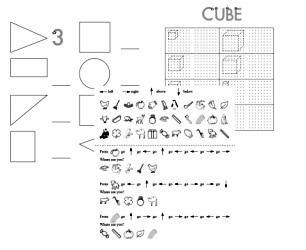Kindergarten Geometry Printables, Worksheets, and Lessons

Kindergarten Geometry Mixed Review Book

Kindergarten Geometry: Tracing, Drawing, and Counting Shapes
Circles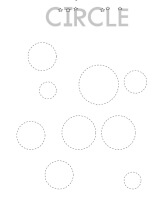Trace Circles (LARGE Circles - few per page) Trace and Count Circles (LARGE Circles - few per page) Trace Circles (a little smaller - more circles per page) Trace and Count Circles (a little smaller - more circles per page) Trace Circles (very small circles) Trace and Count Circles (very small circles)

Rectangles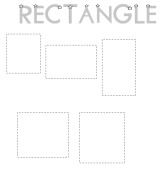Trace Rectangles (LARGE Rectangles - few per page) Trace and Count Rectangles (LARGE Rectangles - few per page) Trace Rectangles (a little smaller) Trace and Count Rectangles (a little smaller) Trace Rectangles (very small) Trace and Count Rectangles (very small)

Squares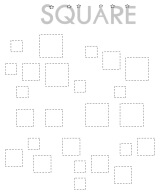Trace Squares (LARGE Squares - few per page) Trace and Count Squares (LARGE Squares - few per page) Trace Squares (a little smaller) Trace and Count Squares (a little smaller) Trace Squares (very small) Trace and Count Squares (very small)

Triangles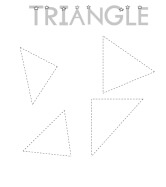Trace Triangles (LARGE Triangles - few per page) Trace and Count Triangles (LARGE Triangles - few per page) Trace Triangles (a little smaller) Trace and Count Triangles (a little smaller) Trace Triangles (very small) Trace and Count Triangles (very small)

Circles, Squares, Rectangles, and Triangles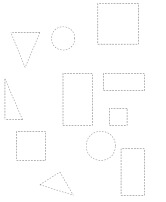Trace Circles, Squares, Rectangles, and Triangles (LARGE) Trace and Count Circles, Squares, Rectangles, and Triangles (LARGE) Trace Circles, Squares, Rectangles, and Triangles (a little smaller) Trace and Count Circles, Squares, Rectangles, and Triangles (a little smaller) Trace Circles, Squares, Rectangles, and Triangles (very small) Trace and Count Circles, Squares, Rectangles, and Triangles (very small)

Kindergarten Geometry: Understanding Shape Words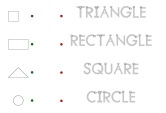Match Shapes with Spelling of Word

Kindergarten Geometry: Tracing and then Copying a Shape
Larger Shapes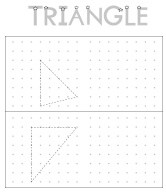Circles: Trace and Copy Circles Rectangles: Trace and Copy Rectangles Squares: Trace and Copy Squares Triangles: Trace and Copy Triangles

Smaller Shapes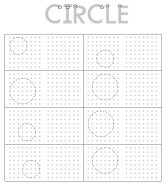Circles: Trace and Copy Circles Rectangles: Trace and Copy Rectangles Squares: Trace and Copy Squares Triangles: Trace and Copy Triangles

Locations: Right, Left, Below, and Above
Easier - Help on Top and Larger Images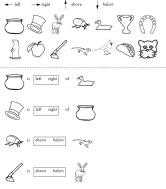Multiple Choice: Left and Right Multiple Choice: Above and Below Multiple Choice: Left, Right, Above and Below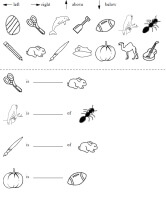Fill-in: Left and Right Fill-in: Above and Below Fill-in: Left, Right, Above and Below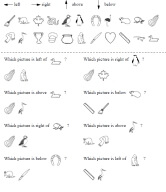Picture Multiple Choice: Left and Right Picture Multiple Choice: Above and Below Picture Multiple Choice: Left, Right, Above and Below

Harder - No Help on Top and Smaller Images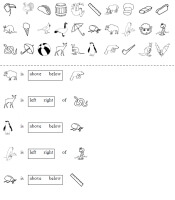Multiple Choice: Left and Right Multiple Choice: Above and Below Multiple Choice: Left, Right, Above and Below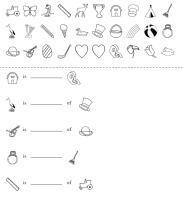Fill-in: Left and Right Fill-in: Above and Below Fill-in: Left, Right, Above and Below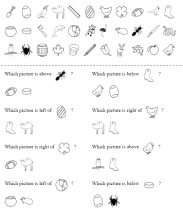Picture Multiple Choice: Left and Right Picture Multiple Choice: Above and Below Picture Multiple Choice: Left, Right, Above and Below

Following Directions - Locations: Right, Left, Below, and Above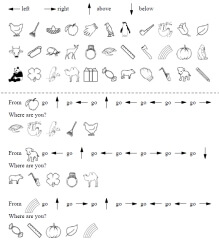Following Directions

Solid Figures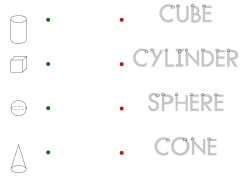Matching Solid Figures with Traceable Words (rectangular prism not included)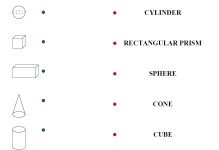Matching Solid Figures with Words

Shapes - Counting Number of Sides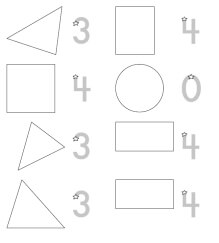Shapes - Counting Number of Sides (traceable answer given for each)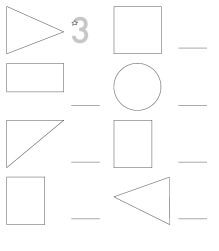Shapes - Counting Number of Sides (traceable answer for first shape only)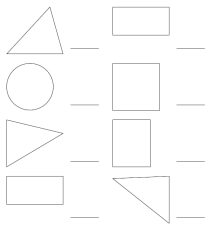Shapes - Counting Number of Sides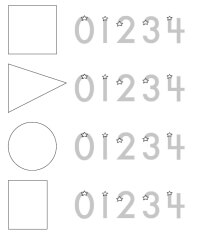Shapes - Counting Number of Sides - Circle the Answer

Draw Shapes and Trace Word - Circle, Rectangle, Square, Triangle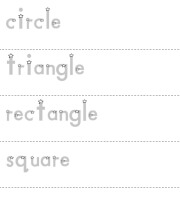Draw Shapes and Trace Word (uppercase words) Draw Shapes and Trace Word (lowercase words)

Complete Shapes - Circle, Rectangle, Square, Triangle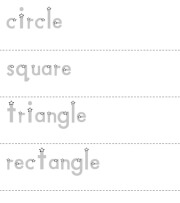Complete the Shapes

Matching Solid Figures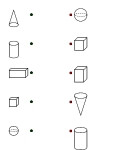Match the Solid Figures

Tracing and then Drawing Solid Figures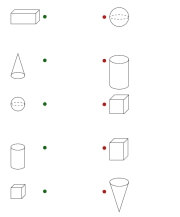Trace and Draw a Cube (larger cubes) Trace and Draw a Cube (smaller cubes)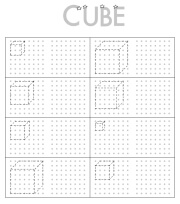Trace and Draw a Rectangular Prism (larger rectangular prisms) Trace and Draw a Rectangular Prism (smaller rectangular prisms)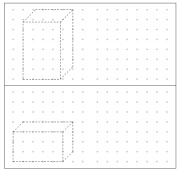Trace and Draw a Cone (larger cones) Trace and Draw a Cone (smaller cones)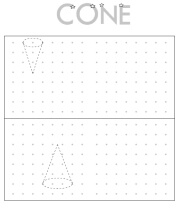Trace and Draw a Cylinder (larger cylinders) Trace and Draw a Cylinder (smaller cylinders)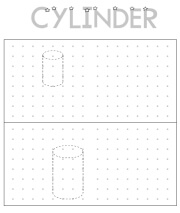Trace and Draw a Sphere (larger spheres) Trace and Draw a Sphere (smaller spheres)

Left and Right, Above and Below

Symmetry and Lines of Symmetry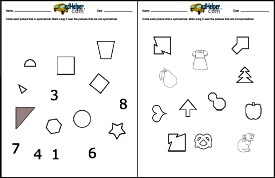Symmetry and Lines of Symmetry

Shape Mazes

Ordered Pairs Printables

Have a suggestion or would like to leave feedback?